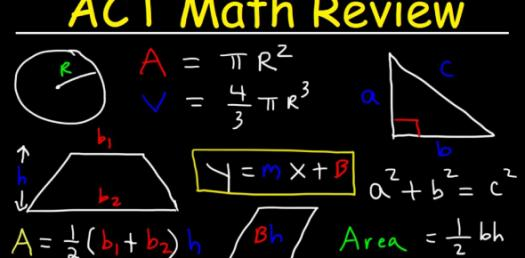# Act 5-in-5: Trivia Math Questions! Quiz

Approved & Edited by ProProfs Editorial Team
The editorial team at ProProfs Quizzes consists of a select group of subject experts, trivia writers, and quiz masters who have authored over 10,000 quizzes taken by more than 100 million users. This team includes our in-house seasoned quiz moderators and subject matter experts. Our editorial experts, spread across the world, are rigorously trained using our comprehensive guidelines to ensure that you receive the highest quality quizzes.
| By Melacat
M
Melacat
Community Contributor
Quizzes Created: 4 | Total Attempts: 2,912
Questions: 5 | Attempts: 769Settings• 1.

• 2.
• A.

- 9

• B.

- 6

• C.

- 3

• D.

0

• E.

3

D. 0
• 3.

### If 9x - 41 = 2(2x - 3), then x = ?

• A.

5

• B.

7

• C.

9

• D.

11

• E.

13

B. 7
Explanation
To solve the equation 9x - 41 = 2(2x - 3), we can start by distributing the 2 on the right side of the equation, giving us 9x - 41 = 4x - 6. Next, we can combine like terms by subtracting 4x from both sides, resulting in 5x - 41 = -6. To isolate the variable x, we can add 41 to both sides, giving us 5x = 35. Finally, by dividing both sides by 5, we find that x = 7.

Rate this question:

• 4.

### To make space for new merchandise, a clothing store offered a 15% discount on all current stock.  What was the sale price of a jacket that regularly sells for \$24?

• A.

\$9.00

• B.

\$20.40

• C.

\$21.60

• D.

\$22.50

• E.

\$23.85

B. \$20.40
Explanation
The sale price of the jacket can be calculated by subtracting the discount from the regular price. The discount is 15% of \$24, which is \$3.60. Subtracting \$3.60 from \$24 gives us \$20.40, which is the sale price of the jacket.

Rate this question:

• 5.
• A.

30 degrees

• B.

50 degrees

• C.

60 degrees

• D.

120 degrees

• E.

Cannot be determined from the given information.

C. 60 degrees
• 6.
• A.

9.0

• B.

12.0

• C.

79.5

• D.

153.0

• E.

1689.0Back to top